NEET  >  31 Year NEET Previous Year Questions: Motion in a Straight Line - 2

# 31 Year NEET Previous Year Questions: Motion in a Straight Line - 2

Test Description

## 30 Questions MCQ Test Physics Class 11 | 31 Year NEET Previous Year Questions: Motion in a Straight Line - 2

31 Year NEET Previous Year Questions: Motion in a Straight Line - 2 for NEET 2023 is part of Physics Class 11 preparation. The 31 Year NEET Previous Year Questions: Motion in a Straight Line - 2 questions and answers have been prepared according to the NEET exam syllabus.The 31 Year NEET Previous Year Questions: Motion in a Straight Line - 2 MCQs are made for NEET 2023 Exam. Find important definitions, questions, notes, meanings, examples, exercises, MCQs and online tests for 31 Year NEET Previous Year Questions: Motion in a Straight Line - 2 below.
Solutions of 31 Year NEET Previous Year Questions: Motion in a Straight Line - 2 questions in English are available as part of our Physics Class 11 for NEET & 31 Year NEET Previous Year Questions: Motion in a Straight Line - 2 solutions in Hindi for Physics Class 11 course. Download more important topics, notes, lectures and mock test series for NEET Exam by signing up for free. Attempt 31 Year NEET Previous Year Questions: Motion in a Straight Line - 2 | 30 questions in 60 minutes | Mock test for NEET preparation | Free important questions MCQ to study Physics Class 11 for NEET Exam | Download free PDF with solutions
 1 Crore+ students have signed up on EduRev. Have you?
31 Year NEET Previous Year Questions: Motion in a Straight Line - 2 - Question 1

### A car is moving along a straight road with a uniform acceleration. It passes through two points P and Q separated by a distance with velocity 30 km/h and 40 km/h respectively. The velocity of the car midway between P and Q is 

Detailed Solution for 31 Year NEET Previous Year Questions: Motion in a Straight Line - 2 - Question 1

Let PQ = x , then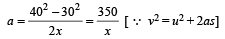Also, velocity at mid point is given by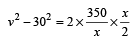This gives​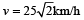31 Year NEET Previous Year Questions: Motion in a Straight Line - 2 - Question 2

### A train of 150 metre long is going towards north direction at a speed of 10 m/s . A parrot flies at the speed of 5 m/s towards south direction parallel to the railway track. The time taken by the parrot to cross the train is [1988, 1992]

Detailed Solution for 31 Year NEET Previous Year Questions: Motion in a Straight Line - 2 - Question 2

Relative velocity of parrot w.r.t the train = 10 – (–5) = 15 ms–1.
Time taken by parrot to cross the train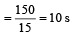31 Year NEET Previous Year Questions: Motion in a Straight Line - 2 - Question 3

### What will be the ratio of the distances moved by a freely falling body from rest in 4th and 5th seconds of journey? 

Detailed Solution for 31 Year NEET Previous Year Questions: Motion in a Straight Line - 2 - Question 3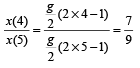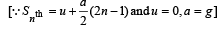31 Year NEET Previous Year Questions: Motion in a Straight Line - 2 - Question 4

A car covers the first half of the distance between two places at 40 km/h and other half at 60 km/h.The average speed of the car is 

Detailed Solution for 31 Year NEET Previous Year Questions: Motion in a Straight Line - 2 - Question 4

Total distance = s;

Total time taken  =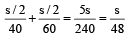∴ Average speed =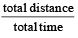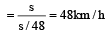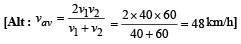31 Year NEET Previous Year Questions: Motion in a Straight Line - 2 - Question 5

A bus travelling the first one third distance at a speed of 10 km/h, the next one third at 20 km/h and the last one-third at 60 km/h. The average speed of the bus is 

Detailed Solution for 31 Year NEET Previous Year Questions: Motion in a Straight Line - 2 - Question 5

Let total distance be x

It covered
x/3 at 10
x/3 at 20
x/3 at 60
Times taken respectively are { d / t }
x / 30
x / 60
x / 180 respectively

total time = x/30 + x /60 + x/180
= 10x / 180
= x / 18

Average speed of bus (avg s )
= total distance / total time

avg s = x / {x/18}
avg s = 18 km / h

31 Year NEET Previous Year Questions: Motion in a Straight Line - 2 - Question 6

A car moves a distance of 200 m. It covers the first half of the distance at speed 40 km/h and the second half of distance at speed v. The average speed is 48 km/h. Find the value of v

Detailed Solution for 31 Year NEET Previous Year Questions: Motion in a Straight Line - 2 - Question 6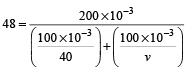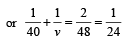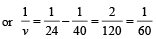or  v = 60 km/h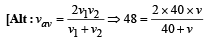⇒ v = 60 km/h

31 Year NEET Previous Year Questions: Motion in a Straight Line - 2 - Question 7

A body dropped from top of a tower fall through 40 m during the last two seconds of its fall. The height of tower is (g = 10 m/s2) 

Detailed Solution for 31 Year NEET Previous Year Questions: Motion in a Straight Line - 2 - Question 7

Let the body fall through the height of tower in t seconds. From,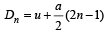we have, total distance travelled in last 2 seconds of fall is

D = Dt+D(t -1)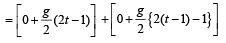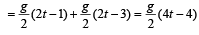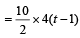or,  40 = 20 (t – 1) or t = 2 + 1 = 3s

Distance travelled in t seconds is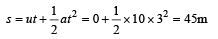31 Year NEET Previous Year Questions: Motion in a Straight Line - 2 - Question 8

Which of the following curve does not represent motion in one dimension? 

Detailed Solution for 31 Year NEET Previous Year Questions: Motion in a Straight Line - 2 - Question 8

In one dimensional motion, the body can have at a time one velocity but not two values of velocities.

31 Year NEET Previous Year Questions: Motion in a Straight Line - 2 - Question 9

A body starts from rest, what is the ratio of the distance travelled by the body during the 4th and 3rd seconds ? 

Detailed Solution for 31 Year NEET Previous Year Questions: Motion in a Straight Line - 2 - Question 9

u = 0 m/s
S = u + (1/2)a(2n - 1)
S₄ = 0 + 1/2(2×4 - 1)
= 7a/2
S₃ = 0 + (1/2) a (2×3 - 1)
= 5a/2
Ratio, S₄/S₃ = 7a/2 ÷ 5a/2
=7/5

31 Year NEET Previous Year Questions: Motion in a Straight Line - 2 - Question 10

A car accelerates from rest at a constant rate α for some time, after which it decelerates at a constant rate β and comes to rest. If the total time elapsed is t, then the maximum velocity acquired by the car is 

Detailed Solution for 31 Year NEET Previous Year Questions: Motion in a Straight Line - 2 - Question 10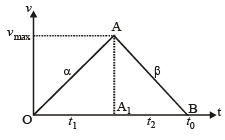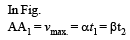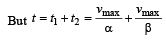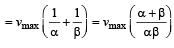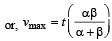31 Year NEET Previous Year Questions: Motion in a Straight Line - 2 - Question 11

The displacement time graph of a moving particle is shown below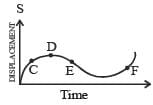The instantaneous velocity of the particle is negative at the point 

Detailed Solution for 31 Year NEET Previous Year Questions: Motion in a Straight Line - 2 - Question 11

At E, the slope of the curve is negative.

31 Year NEET Previous Year Questions: Motion in a Straight Line - 2 - Question 12

A particle moves along a straight line such that its displacement at any time t is given by s = (t3 – 6t2 + 3t + 4) metres The velocity when the acceleration is zero is

Detailed Solution for 31 Year NEET Previous Year Questions: Motion in a Straight Line - 2 - Question 12

Velocity,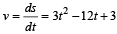Acceleration,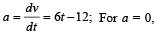we have, 0 = 6 t – 12 or t = 2s.

Hence, at t = 2 s the velocity will be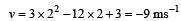31 Year NEET Previous Year Questions: Motion in a Straight Line - 2 - Question 13

A body is thrown vertically upward from the ground. It reaches a maximum height of 20 m in 5 sec. After what time, it will reach the ground from its maximum height position? 

Detailed Solution for 31 Year NEET Previous Year Questions: Motion in a Straight Line - 2 - Question 13

hmax = 20 m and t = 5 sec. Time taken by the body to reach the ground from some height is the same as taken to reach that height.
Therefore, time to reach the ground from its maximum height is 5 sec.

31 Year NEET Previous Year Questions: Motion in a Straight Line - 2 - Question 14

A stone released with zero velocity from the top of a tower, reaches the ground in 4 sec. The height of the tower is (g = 10m / s2 ) 

Detailed Solution for 31 Year NEET Previous Year Questions: Motion in a Straight Line - 2 - Question 14

Initial velocity (u) = 0; Time (t) = 4 sec and gravitational acceleration (g) = 10 m/s2.
Height of tower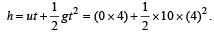= 80 m.

31 Year NEET Previous Year Questions: Motion in a Straight Line - 2 - Question 15

Three different objects of masses m1, m2 and m3 are allowed to fall from rest and from the same point O along three different frictionless paths.The speeds of the three objects on reaching the ground will be in the ratio of 

Detailed Solution for 31 Year NEET Previous Year Questions: Motion in a Straight Line - 2 - Question 15

The speed of an object, falling freely due to gravity, depends only on its height and not on its mass. Since the paths are frictionless and all the objects fall through the same height, therefore, their speeds on reaching the ground will be in the ratio of 1 : 1 : 1.

31 Year NEET Previous Year Questions: Motion in a Straight Line - 2 - Question 16

The water drops fall at regular intervals from a tap 5 m above the ground. The third drop is leaving the tap at an instant when the first drop touches the ground. How far above the ground is the second drop at that instant ? (Take g = 10 m/s2)

Detailed Solution for 31 Year NEET Previous Year Questions: Motion in a Straight Line - 2 - Question 16

Height of tap = 5m and (g) = 10 m/sec2.
For the first drop, 5 =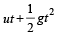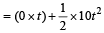= 5t2 or t2 = 1 or t = 1 sec.
It means that the third drop leaves after one second of the first drop. Or, each drop leaves after every 0.5 sec.
Distance covered by the second drop in  0.5 sec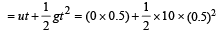= 1.25 m.
Therefore, distance of the second drop above the ground = 5 – 1.25 = 3.75 m.

31 Year NEET Previous Year Questions: Motion in a Straight Line - 2 - Question 17

If a ball is thrown vertically upwards with a velocity of 40 m/s, then velocity of the ball after two seconds will be (g = 10 m/s2) 

Detailed Solution for 31 Year NEET Previous Year Questions: Motion in a Straight Line - 2 - Question 17

Initial velocity (u) = 40 m/s
Acceleration a = –g m/s2 = –10 m/s2
Time = 2 seconds
By Ist equation of motion, v = u + at
v = 40 – 10 (2) = 20 m/s

31 Year NEET Previous Year Questions: Motion in a Straight Line - 2 - Question 18

The displacement of a particle varies with time (t) as: s = at2 – bt3. The acceleration of particle is zero, at time T equal to

Detailed Solution for 31 Year NEET Previous Year Questions: Motion in a Straight Line - 2 - Question 18

s = at2 – bt3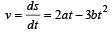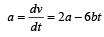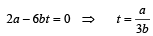31 Year NEET Previous Year Questions: Motion in a Straight Line - 2 - Question 19

If a car at rest accelerates uniformly to a speed of 144 km/h in 20 s, it covers a distance of 

Detailed Solution for 31 Year NEET Previous Year Questions: Motion in a Straight Line - 2 - Question 19

Initial velocity of car (u) = 0
Final velocity of car (v) = 144 km/hr = 40 m/s
Time taken = 20 s
We know that, v = u + at 40 = a × 20 ⇒ a = 2 m/s2
Also, v2 – u2 = 2as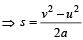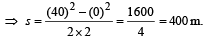31 Year NEET Previous Year Questions: Motion in a Straight Line - 2 - Question 20

A car moving with a speed of 40 km/h can be stopped by applying brakes at least after 2 m. If the same car is moving with a speed of 80 km/h, what is the minimum stopping distance? 

Detailed Solution for 31 Year NEET Previous Year Questions: Motion in a Straight Line - 2 - Question 20

v2 – u2 = 2as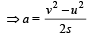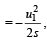where u1 = 40 km/h

For same retarding force s ∝ u2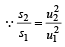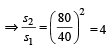∴ s2 =   4s =   8mIf

F is retarding force and s the stopping distance, then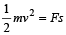For same retarding force, s α v2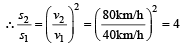∴ s2 = 4s1 = 4 x 2= 8m

31 Year NEET Previous Year Questions: Motion in a Straight Line - 2 - Question 21

The displacement of a particle is represented by the following equation : s = 3t3 + 7t2 + 5t+8 where s is in metre and t in second. The acceleration of the particle at t = 1s is 

Detailed Solution for 31 Year NEET Previous Year Questions: Motion in a Straight Line - 2 - Question 21

Displacement s = 3t3 + 7t2 + 5t +8;

Velocity =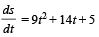Acceleration =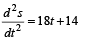Acceleration at (t = 1s) = 18×1+14 = 18 + 14 = 32 m/s2

31 Year NEET Previous Year Questions: Motion in a Straight Line - 2 - Question 22

A stone is thrown vertically upwards with kinetic energy K. The kinetic energy at the highest point is 

Detailed Solution for 31 Year NEET Previous Year Questions: Motion in a Straight Line - 2 - Question 22

At heigh est point, velocity is zero.
Hence, Kinetic energy is zero.

31 Year NEET Previous Year Questions: Motion in a Straight Line - 2 - Question 23

If a ball is thrown vertically upwards with speed u, the distance covered during the last t seconds of its ascent is 

Detailed Solution for 31 Year NEET Previous Year Questions: Motion in a Straight Line - 2 - Question 23

Let body takes T sec to reach maximum height.

Then v = u – gT
v = 0, at highest point.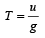...................(1]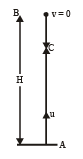Velocity attained by body in (T – t) sec

v = u – g (T – t)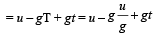or v = gt ...................(2)

∴   Distance travelled in last t sec of its ascent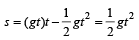31 Year NEET Previous Year Questions: Motion in a Straight Line - 2 - Question 24

A man throws balls with the same speed vertically upwards one after the other at an interval of 2 seconds. What should be the speed of the throw so that more than two balls are in the sky at any time? [Given g = 9.8 m/s2] 

Detailed Solution for 31 Year NEET Previous Year Questions: Motion in a Straight Line - 2 - Question 24

Let the required speed of throw be u ms–1.
Then time taken to reach maximum height,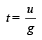For two balls to remain in air at any time, t must be greater than 2.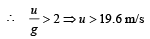31 Year NEET Previous Year Questions: Motion in a Straight Line - 2 - Question 25

The displacement x of a particle varies with time t as x = ae-αt + beβt, where a, b, α and β are positive constants. The velocity of the particle will [2 00 5]

Detailed Solution for 31 Year NEET Previous Year Questions: Motion in a Straight Line - 2 - Question 25

Given x = ae–αt + beβt

Velocity,  v =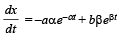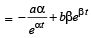i.e., go on increasing with time.

31 Year NEET Previous Year Questions: Motion in a Straight Line - 2 - Question 26

A ball is thrown ver tically upward. It has a speed of 10 m/sec when it has reached one half of its maximum height. How high does th e ball rise? [2001, 2005] Take g = 10 m/s2.

Detailed Solution for 31 Year NEET Previous Year Questions: Motion in a Straight Line - 2 - Question 26

For part AB
From 3rd equation of motion v2 = u2 – 2gH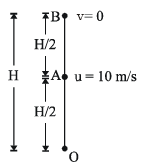0  =  u2 – 2g(H/2) = u2 – gH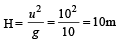31 Year NEET Previous Year Questions: Motion in a Straight Line - 2 - Question 27

A  particle moves along a straight line OX. At a time t (in seconds) the distance x (in metres) of the particle from O is given by x = 40 + 12t – t3. How long would the particle travel before coming to rest? 

Detailed Solution for 31 Year NEET Previous Year Questions: Motion in a Straight Line - 2 - Question 27

At t = 0, the particle is at, let's say x distance, from O;
then putting t = 0 in the given displacement-time equation we get;

► x = 40 + 12(0) − (0)3 = 40m

Particle comes to rest that means the velocity of the particle becomes zero after travelling certain displacement; let's say the time be t.

then after differentiating the given displacement−time equation wrt. time we get the velocity−time equation

► v = 12 − 3t2

at time t = t (the time when the particle comes to rest ): v = 0;
► 12 − 3t= 0;
► t = 2s

Then, at t = 2s we are at, let's say x′ distance from O;

put this value of t (=2) in given displacement-time equation, we get;

►  x′ = 40 + 12 (2) − (2)3
= 56m

Further;
We have seen that the particle started his journey when it is at 40m from point O.
And came to rest at 56m from point O.
then the particle travelled a distance of:

► 56 − 40 = 16m.

31 Year NEET Previous Year Questions: Motion in a Straight Line - 2 - Question 28

Two bodies, A (of mass 1 kg) and B (of mass 3 kg), are dropped from heights of 16m and 25m, respectively. The ratio of the time taken by them to reach the ground is 

Detailed Solution for 31 Year NEET Previous Year Questions: Motion in a Straight Line - 2 - Question 28

Let t1 & t2 be the time taken by A and B respectively to reach the ground then from the formula,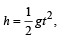For first body,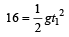For second body,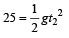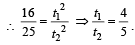31 Year NEET Previous Year Questions: Motion in a Straight Line - 2 - Question 29

The position x of a particle with respect to time t along x-axis is given by x = 9t2 – t3 where x is in metres and t in second. What will be the position of this particle when it achieves maximum speed along the +ve x direction? 

Detailed Solution for 31 Year NEET Previous Year Questions: Motion in a Straight Line - 2 - Question 29

Speed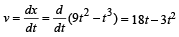For maximum speed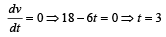⇒ xmax = 81 - 27= 54

31 Year NEET Previous Year Questions: Motion in a Straight Line - 2 - Question 30

A car moves from X to Y with a uniform speed vu and returns to Y with a uniform speed vd. The average speed for this round trip is 

Detailed Solution for 31 Year NEET Previous Year Questions: Motion in a Straight Line - 2 - Question 30

Average speed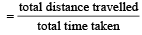Let s be the distance from X to Y.

∴ Average speed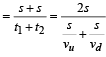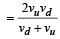## Physics Class 11

127 videos|464 docs|210 tests
 Use Code STAYHOME200 and get INR 200 additional OFF Use Coupon Code
Information about 31 Year NEET Previous Year Questions: Motion in a Straight Line - 2 Page
In this test you can find the Exam questions for 31 Year NEET Previous Year Questions: Motion in a Straight Line - 2 solved & explained in the simplest way possible. Besides giving Questions and answers for 31 Year NEET Previous Year Questions: Motion in a Straight Line - 2, EduRev gives you an ample number of Online tests for practice

## Physics Class 11

127 videos|464 docs|210 tests

### How to Prepare for NEET

Read our guide to prepare for NEET which is created by Toppers & the best Teachers